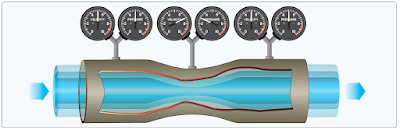# Theories in the Production of Lift

In order to achieve flight in a machine that is heavier than air, there are several obstacles we must overcome. One of those obstacles, discussed previously, is the resistance to movement called drag. The most challenging obstacle to overcome in aviation, however, is the force of gravity. A wing moving through air generates the force called lift, also previously discussed. Lift from the wing that is greater than the force of gravity, directed opposite to the direction of gravity, enables an aircraft to fly. Generating this force called lift is based on some important principles, Newton's basic laws of motion, and Bernoulli's principle of differential pressure.

## Newton’s Basic Laws of Motion

The formulation of lift has historically been an adaptation over the past few centuries of basic physical laws. These laws, although seemingly applicable to all aspects of lift, do not explain how lift is formulated. In fact, one must consider the many airfoils that are symmetrical, yet produce significant lift.

The fundamental physical laws governing the forces acting upon an aircraft in flight were adopted from postulated theories developed before any human successfully flew an aircraft. The use of these physical laws grew out of the Scientific Revolution, which began in Europe in the 1600s. Driven by the belief the universe operated in a predictable manner open to human understanding, many philosophers, mathematicians, natural scientists, and inventors spent their lives unlocking the secrets of the universe. One of the most well-known was Sir Isaac Newton, who not only formulated the law of universal gravitation, but also described the three basic laws of motion.

Newton’s First Law: “Every object persists in its state of rest or uniform motion in a straight line unless it is compelled to change that state by forces impressed on it.”

This means that nothing starts or stops moving until some outside force causes it to do so. An aircraft at rest on the ramp remains at rest unless a force strong enough to overcome its inertia is applied. Once it is moving, its inertia keeps it moving, subject to the various other forces acting on it. These forces may add to its motion, slow it down, or change its direction.

Newton’s Second Law: “Force is equal to the change in momentum per change in time. For a constant mass, force equals mass times acceleration.”

When a body is acted upon by a constant force, its resulting acceleration is inversely proportional to the mass of the body and is directly proportional to the applied force. This takes into account the factors involved in overcoming Newton’s First Law. It covers both changes in direction and speed, including starting up from rest (positive acceleration) and coming to a stop (negative acceleration or deceleration).

Newton’s Third Law: “For every action, there is an equal and opposite reaction.”

In an airplane, the propeller moves and pushes back the air; consequently, the air pushes the propeller (and thus the airplane) in the opposite direction—forward. In a jet airplane, the engine pushes a blast of hot gases backward; the force of equal and opposite reaction pushes against the engine and forces the airplane forward.

## Bernoulli’s Principle of Differential Pressure

A half-century after Newton formulated his laws, Daniel Bernoulli, a Swiss mathematician, explained how the pressure of a moving fluid (liquid or gas) varies with its speed of motion. Bernoulli’s Principle states that as the velocity of a moving fluid (liquid or gas) increases, the pressure within the fluid decreases. This principle explains what happens to air passing over the curved top of the airplane wing.

A practical application of Bernoulli’s Principle is the venturi tube. The venturi tube has an air inlet that narrows to a throat (constricted point) and an outlet section that increases in diameter toward the rear. The diameter of the outlet is the same as that of the inlet. The mass of air entering the tube must exactly equal the mass exiting the tube. At the constriction, the speed must increase to allow the same amount of air to pass in the same amount of time as in all other parts of the tube. When the air speeds up, the pressure also decreases. Past the constriction, the airflow slows and the pressure increases. [Figure]Air pressure decreases in a venturi tube

Since air is recognized as a body, and it is understood that air will follow the above laws, one can begin to see how and why an airplane wing develops lift. As the wing moves through the air, the flow of air across the curved top surface increases in velocity creating a low-pressure area. Although Newton, Bernoulli, and hundreds of other early scientists who studied the physical laws of the universe did not have the sophisticated laboratories available today, they provided great insight to the contemporary viewpoint of how lift is created.

RELATED POSTS